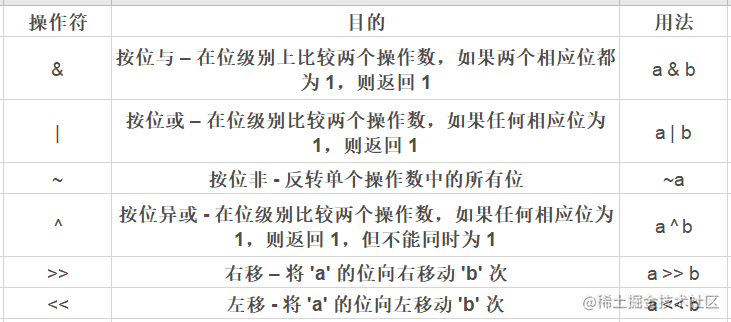# Python 运算符学习教程｜Python 主题月

## 目录

### Python 中的运算符是什么？

``````>>> 7%4
3

### 算术运算符

+加法 - 两个操作数的总和a+b

*乘法——两个操作数的乘积a*b
/Float除法 - 两个操作数的商a/b
//Floor除法 – 两个操作数的商（没有小数部分）a//b
%模数 – 'a' 除以 'b' 后的整数余数。a%b
**指数 – 'a' 本身的乘积 'b' 次（a 的 b 次方）a**b

``````a=7
b=3

print('加 : ', a+b)
print('减 : ', a-b)
print('乘 : ', a*b)
print('除 (float) : ', a/b)
print('除 (floor) : ', a//b)
print('模数 : ', a%b)
print('指数 : ', a**b)

``````加 :  10

### 比较运算符

>大于 - 如果左操作数大于右操作数，则返回 true。a>b
<小于 - 如果左操作数小于右操作数，则返回 true。a<b
==等于 - 如果两个操作数相等，则返回 true。a==b
!=不等于 - 如果两个操作数不相等，则返回 true。a!=b
>=大于或等于 - 如果左操作数大于或等于右操作数，则返回 true。a>=b
<=小于或等于 - 如果左操作数小于或等于右操作数，则返回 true。a<=b

``````a=9
b=8

print('a > b is',a>b)

print('a < b is',a<b)

print('a == b is',a==b)

print('a != b is',a!=b)

print('a >= b is',a>=b)

print('a <= b is',a<=b)

``````a > b is True
a < b is False
a == b is False
a != b is True
a >= b is True
a <= b is False

### 逻辑运算符

and如果“a”为假，则为“a”，否则为“b”a and b
or如果“a”为假，则为“b”，否则为“a”a or b
not如果“a”为假，则为真，否则为假not a

``````a=7
b=4

# 结果: a and b is 4
print('a and b is',a and b)

# 结果: a or b is 7
print('a or b is',a or b)

# 结果: not a is False
print('not a is',not a)

``````a and b is 4
a or b is 7
not a is False

### 按位运算符``````a=4
b=6

#Bitwise AND: The result of 'a & b' is 4
print('a & b is',a & b)

Output-

``````a & b is 4

`````` 0 0 0 0 0 1 0 0 &
0 0 0 0 0 1 1 0
------------------
0 0 0 0 0 1 0 0（数字4的二进制表示）

### 赋值运算符

=a=4a=4
+=a+=4a=a+4
-=a-=4a=a-4
*=a*=4a=a*4
/=a/=4a=a/4
%=%=4a=a%4
**=**=4a=a**4
&=a&=4a=a&4
=a=4
^=^=4a=a^4
`>>=`a>>=4a=a>>4
<<=一<<=4a=<<4

### 高级 Python 运算符

Python 还捆绑了一些用于特殊用途的运算符。这些被称为高级 Python 运算符，如身份运算符或成员运算符。

#### 身份运算符

isTrue – 如果两个操作数都指向同一个对象，否则为 Falsea 是 b（如果 id(a) 和 id(b) 相同则为真）
is notTrue – 如果操作数引用不同的对象，否则为 Falsea 不是 b（如果 id(a) 和 id(b) 不同则为真）

``````# 使用'is'身份运算符
a = 7
if (type(a) is int):
print("true")
else:
print("false")

# 使用“```
is not
```”身份运算符
b = 7.5
if (type(b) is not int):
print("true")
else:
print("false")

``````真的

#### 成员运算符

inTrue – 如果该值存在于序列中7 in [3, 7, 9]
not inTrue – 如果在序列中未找到该值7 not in [3, 5, 9]

``````# 使用成员运算符

str = 'Python 运算符'
dict = {6:'June',12:'Dec'}

print('P' in str)
print('Python' in str)
print('python' not in str)
print(6 in dict)
print('Dec' in dict)

``````True
True
True
True
False